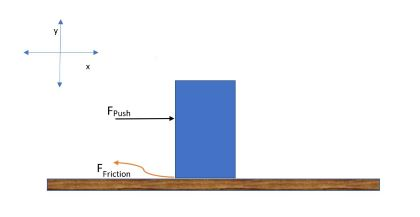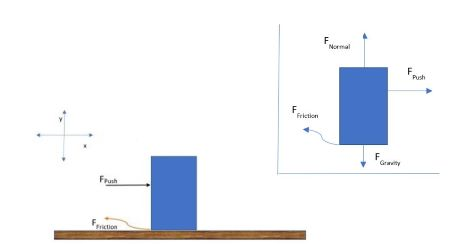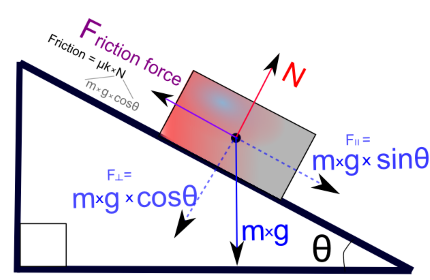# Static Friction Formula & Examples | Coefficient of Static Friction

• Author

Adam has taught physics and test-prep for over twenty years and has a master's degree in physics from Drexel University, an MAS in peace studies and conflict transformation from Basel university, and dual undergrad degrees in physics and biology from SUNY New Paltz.

• Instructor
David Wood

David has taught Honors Physics, AP Physics, IB Physics and general science courses. He has a Masters in Education, and a Bachelors in Physics.

What is static friction? See static friction examples and learn the static friction formula. Compare Static vs. kinetic friction and learn the differences.

Show

## Static Friction Definition

What is static friction? Static friction is the force between two surfaces that keeps them from moving relative to one another. You can get an intuitive sense from everyday experiences like trying to slide a box across a floor and noticing that you have to push it with a certain force before it starts moving. The force pushing against you is the static friction force.It's also fairly intuitive to understand that this force gets larger for more massive objects. The heavier things are, the more force is required to set them into motion. Also, different types of surfaces have different strengths or forces of friction, like rubber on the pavement in comparison to a metal ice skate blade on ice. Therefore, it can be stated that the frictional force is a function of the weight of an object and different types of materials that are in contact with each other.

### What Causes Static Friction?

As noted above, the interaction between the different materials is what causes the static friction force. But what does that really mean?

To better understand this phenomenon, the notion of static friction needs to be broken down into two types of interactions.

• One of those interactions is at the macroscopic level. This is generally referred to as friction, the force that takes place between the ridges of non-smooth surfaces.
• The other is called adhesion, which is the microscopic interaction of the intermolecular forces between the two surfaces. Fundamentally, the interaction that is taking place is the interaction of the valence, or outer shell electrons where the surfaces are making contact, which has to do with how many valence electrons there are, which depends on the types of atoms in the molecules, and how many are exposed on the surface, which depends on the shape and orientation of the molecules at the surface.An error occurred trying to load this video.

Try refreshing the page, or contact customer support.

Coming up next: Molecular Weight: Definition & Formula

### You're on a roll. Keep up the good work!

Replay
Your next lesson will play in 10 seconds
• 0:04 What Is Static Friction?
• 0:49 What Causes Friction?
• 1:20 Inequality for Static Friction
• 3:41 Examples of Static Friction
• 4:00 Lesson Summary
Save Save

Want to watch this again later?

Timeline
Autoplay
Autoplay
Speed Speed## Static Friction Formula

Let's look at a simple example to illustrate how our definition above can be stated mathematically, and we'll show how to find the static friction formula.

According to Newton's First Law, if two things are not in relative motion, then the sum of the forces is equal to zero.

Let's take a look at Figure 1, which illustrates a person trying to push a large bass speaker cabinet across a floor. The inset shows a free-body diagram with the forces in the x and y directions according to the coordinate system in the upper left-hand corner.From the free-body diagram, we can write Newton's Laws for both directions as:

{eq}\sum F_{x}=m_{box}a_{x,box} = F_{push}-F_{friction}=0 {/eq}

{eq}\sum F_{y}=m_{box}a_{y,box} = F_{gravity}-F_{Normal}=0 {/eq}

We're investigating the frictional force FFriction that keeps the speaker cabinet from moving across the floor, which shows up in the 'Fx ' equation. So, what is the static friction formula that tells us what the force is?

As we noted previously, the frictional force FFriction is a function of the weight and the materials that the masses are made of.

If we look at the second equation above, we can see the weight is equal to the Normal force FNormal.

{eq}F_{Gravity} = F_{Normal} {/eq}

So, we can see that FFriction is proportional to FNormal multiplied by some constant that represents the amount of static friction there is between different surfaces and write

{eq}F_{friction}= \mu F_{Normal} {/eq},

where {eq}\mu_{s} {/eq} is the proportionality constant representing the materials and is called the coefficient of static friction.

Let's look at a 2-D situation of a block on an inclined plane where gravity is pulling the box down, but the static friction force keeps it from moving.As we can see from the diagram, the force of gravity can only act on the inclined plane, so we only take the component of the force of gravity along the inclined plane, which is given by Fg,x = mgsin{eq}\theta {/eq}. It is evident from the diagram that the frictional force opposes this motion and is equal in magnitude, but opposite in direction. to Fg,x. Also, FNormal is equal and opposite to Fg,y, which is equal to mgcos{eq}\theta {/eq}.

This gives the frictional force for an object on an inclined plane as:

{eq}F_{Friction} ={\mu_{s}}{F_{Normal}}={\mu_{s}}mgcos\Theta {/eq}

To unlock this lesson you must be a Study.com Member.

### Register to view this lesson

Are you a student or a teacher?